# Introduction to Resonance

Last updated: November 25th, 2019 |

Introduction To Resonance

Last time we talked about how to use electronegativity to find the electron densities in a molecule – and when to ignore formal charge.

However I didn’t mention a second key factor that can  complicate the analysis of electron densities: the presence of double bonds (π bonds). In this post we will see how combining an understanding of dipoles with an understanding of the “best” distribution of pi-electrons in a molecule will give us an overall picture of where electrons are in a molecule.

[Further down the line, an understanding of where the electrons are will help us understand where the molecule will participate in reactions. ]

## 1. Applying Electronegativity Differences To Understand Electron Densities In Molecules With Pi Bonds – Simple Examples

But let’s start with the simple stuff. In many cases, an analysis of electronegativities can give you a very accurate idea of where electron densities are in molecules with π bonds. This can tell you where the “dipoles” are.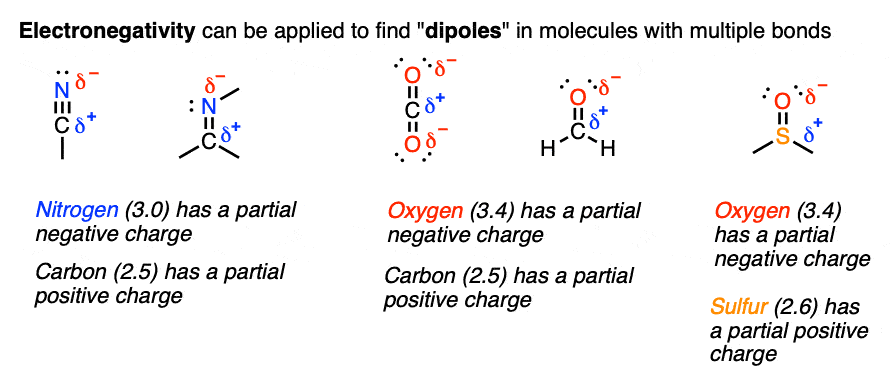Which atom is more electronegative? That’s the one that will be “delta-negative”. Simple enough.

By the way, this model is very effective for finding the reactive sites in these molecules. Here are two examples.

See how the H+ is attracted to the partially negative oxygen, and the HO- is attracted to the partially positive carbon.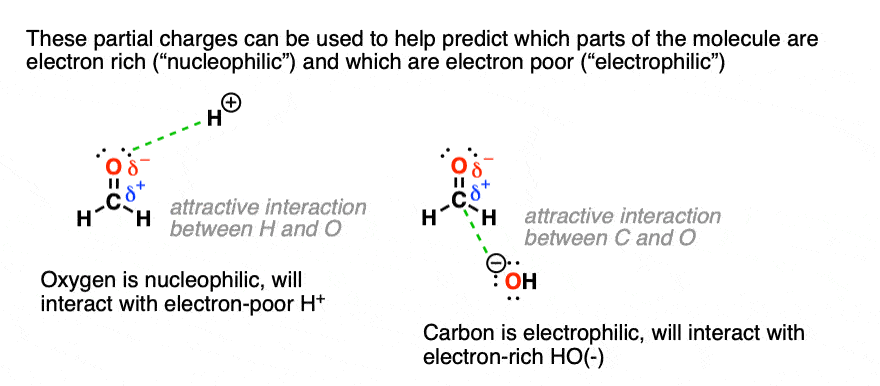Experiments support our prediction that the positively charged acid (i.e. “H+”) will react at the “negative” atom bearing the electrons (oxygen in this case) while negatively charged hydroxide (HO-) will react at the atom bearing the partially positive charge (C in this case).

So far so good.

The problem with our model so far is that it doesn’t allow us to make accurate predictions in some cases.

## 2. Two Cases Where “Applying Electronegativity Differences” Fails To Accurately Predict Electron Density : The Acetate Ion And The Allyl Cation

Part of the experience of being a scientist is watching your very simple, appealing hypotheses/models get ripped to shreds by experimental evidence (or lack thereof).

Look at these two molecules below. The Lewis structure of the first molecule (“acetate ion”) appears to tell us that one oxygen is more negative than the other.

The Lewis structure of the second molecule (the “allyl carbocation”) suggests that one carbon bears the positive charge and the others are neutral.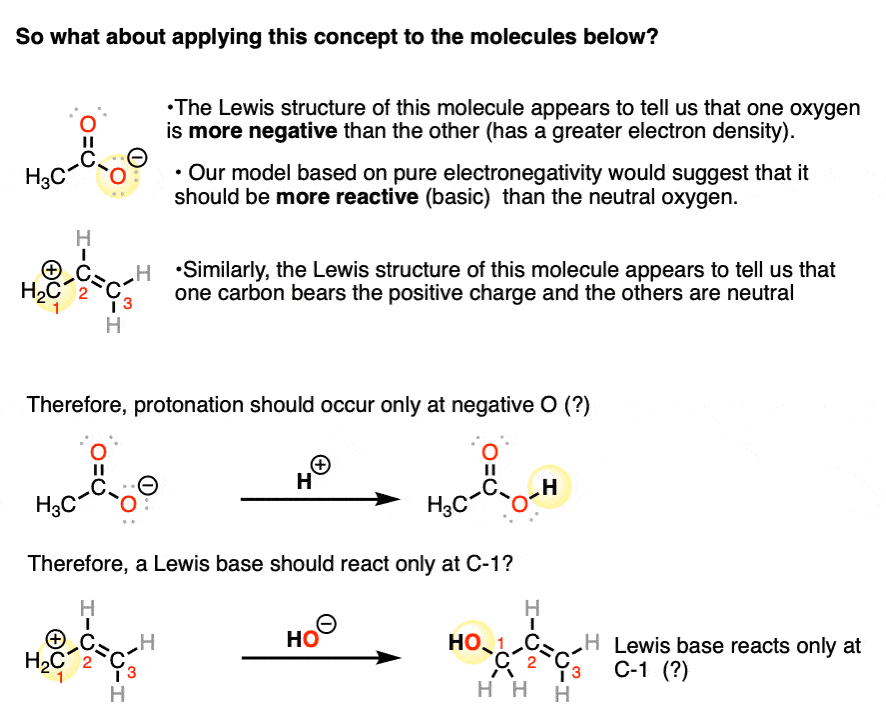We’d predict from this model that the more “negative” oxygen would be more reactive than the neutral oxygen, and likewise for the “positive” and neutral carbons. However, if we test this model by using labelled compounds, we find that our simple model is inconsistent with experiment.

What happens with the first molecule (acetate ion) is that each of the two oxygens is equally reactive with H+.

And in the second example, each of the terminal carbons is equally reactive with nucleophiles.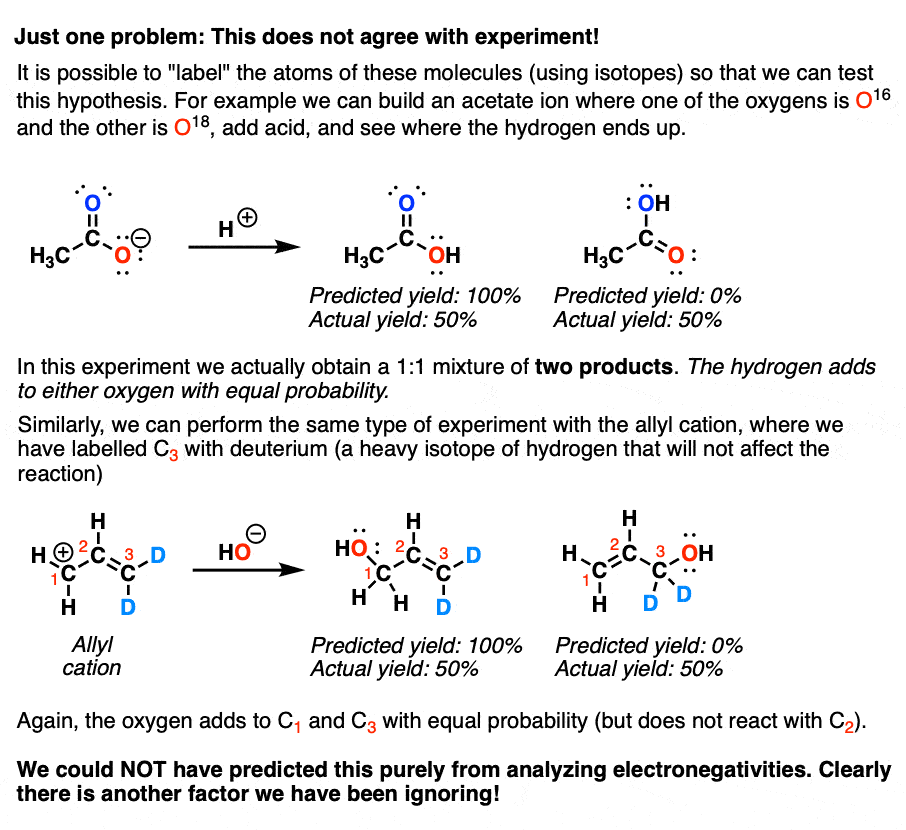Something is clearly lacking in our model. Let’s look at these molecules again.

## 3. It’s Possible To Draw Two Different Lewis Structures For The Acetate Anion And The Allyl Cation

If you look closely it’s possible to draw two different Lewis structures for these molecules.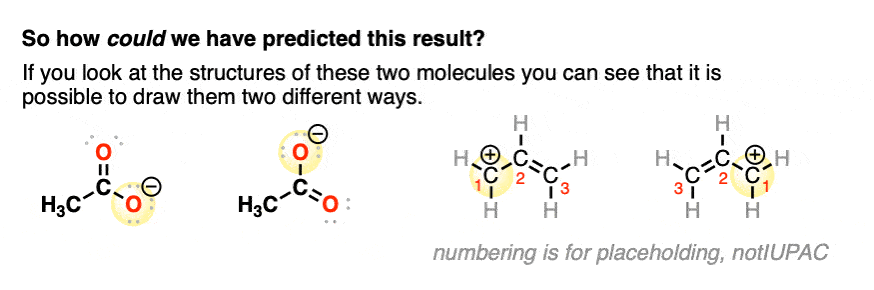From the experiments above, the molecules behave like they are a 1:1 mixture of these two compounds. [They are not, but they behave that way!]

Let’s introduce a new concept: Resonance

## 4. In These Two Cases The “True” Electron Densities Are Reflected By A “Hybrid” of Two Resonance Structures

When we can draw two (or more) forms of the same molecule that differ only in the placement of their electrons, these are called “resonance forms” (or “resonance structures”). We can also say these forms are “in resonance”. Sometimes they are also called resonance “isomers” although this will get a hand-slap in many circles, as they are not technically isomers.

It is tempting (and very wrong!) to think that these  two forms are in “equilibrium” between each other. Avoid this common mistake!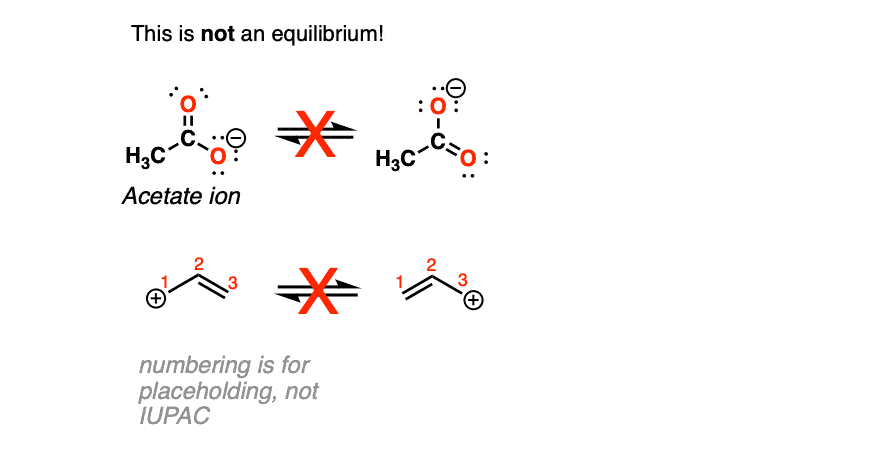Why is this wrong? Because were this true, the bond lengths of the C=O double bond and the C–O single bond in the acetate ion would be different. (1.21 vs. 1.36 Angstrom). However experimental evidence (from X-ray crystal structures) shows us that each bond length is exactly the same (1.26 Angstrom). This observation has been made for countless other resonance isomers as well, such as benzene, carbonate, nitro groups, and many more.

What this means is that the true structure of the acetate ion (and the allyl carbocation) is a “hybrid” of two resonance forms. We use a special arrow to depict the two resonance forms (the double-headed arrow).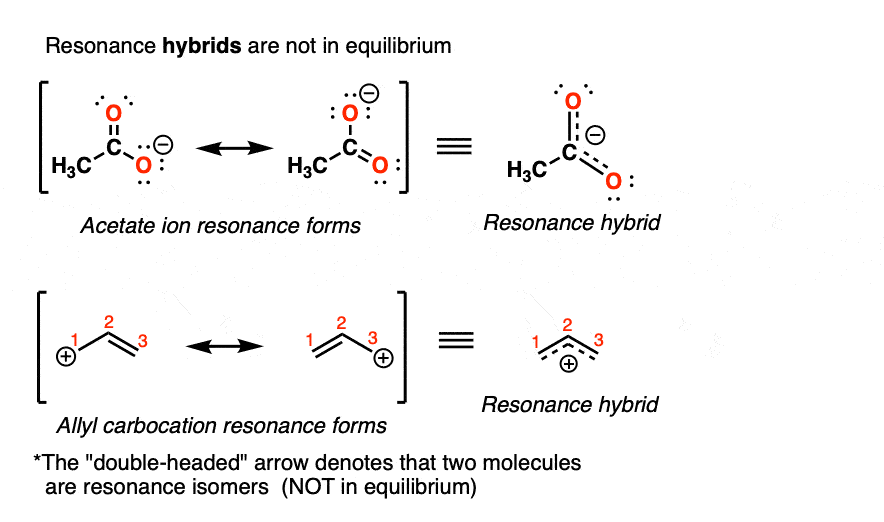What this means is that the formal charge of –1 in the acetate ion is distributed evenly between the two oxygens. And the formal charge of +1 in the allyl carbocation is distributed equally between the two terminal carbons. [Note 1]. As we learned last time, formal charge can be a poor guide to electron densities, so here we see another example where it’s inaccurate.

Again: electron densities are what really matters for understanding the reactivity of a molecule.  Lewis structures and formal charges are imperfect guides to fully describing electron densities in molecules, but they’re so useful in many other contexts that we have to learn to live with their flaws.

However, a combination of understanding dipoles (electronegativity differences) and resonance will complete the picture for us.

Next post we’ll talk about another accounting system we will use to describe the movement of electrons: the curved arrow formalism.

Next Post: Introduction to Curved Arrows (2): Curved Arrows!